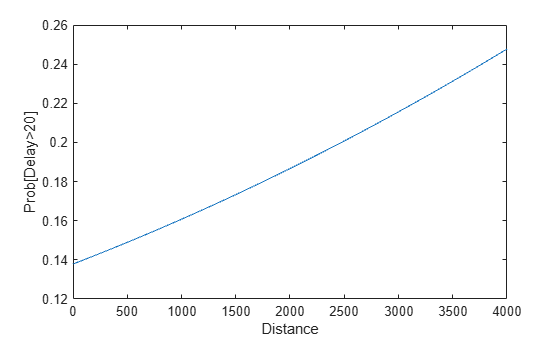# Using MapReduce to Fit a Logistic Regression Model

This example shows how to use `mapreduce` to carry out simple logistic regression using a single predictor. It demonstrates chaining multiple `mapreduce` calls to carry out an iterative algorithm. Since each iteration requires a separate pass through the data, an anonymous function passes information from one iteration to the next to supply information directly to the mapper.

### Prepare Data

Create a datastore using the `airlinesmall.csv` data set. This 12-megabyte data set contains 29 columns of flight information for several airline carriers, including arrival and departure times. In this example, the variables of interest are `ArrDelay` (flight arrival delay) and `Distance` (total flight distance).

```ds = tabularTextDatastore('airlinesmall.csv', 'TreatAsMissing', 'NA'); ds.SelectedVariableNames = {'ArrDelay', 'Distance'};```

The datastore treats `'NA'` values as missing, and replaces the missing values with `NaN` values by default. Additionally, the `SelectedVariableNames` property allows you to work with only the specified variables of interest, which you can verify using `preview`.

`preview(ds)`
```ans=8×2 table ArrDelay Distance ________ ________ 8 308 8 296 21 480 13 296 4 373 59 308 3 447 11 954 ```

### Perform Logistic Regression

Logistic regression is a way to model the probability of an event as a function of another variable. In this example, logistic regression models the probability of a flight being more than 20 minutes late as a function of the flight distance, in thousands of miles.

To accomplish this logistic regression, the map and reduce functions must collectively perform a weighted least-squares regression based on the current coefficient values. The mapper computes a weighted sum of squares and cross product for each block of input data.

Display the map function file.

```function logitMapper(b,t,~,intermKVStore) % Get data input table and remove any rows with missing values y = t.ArrDelay; x = t.Distance; t = ~isnan(x) & ~isnan(y); y = y(t)>20; % late by more than 20 min x = x(t)/1000; % distance in thousands of miles % Compute the linear combination of the predictors, and the estimated mean % probabilities, based on the coefficients from the previous iteration if ~isempty(b) % Compute xb as the linear combination using the current coefficient % values, and derive mean probabilities mu from them xb = b(1)+b(2)*x; mu = 1./(1+exp(-xb)); else % This is the first iteration. Compute starting values for mu that are % 1/4 if y=0 and 3/4 if y=1. Derive xb values from them. mu = (y+.5)/2; xb = log(mu./(1-mu)); end % To perform weighted least squares, compute a sum of squares and cross % products matrix: % (X'*W*X) = (X1'*W1*X1) + (X2'*W2*X2) + ... + (Xn'*Wn*Xn), % where X = [X1;X2;...;Xn] and W = [W1;W2;...;Wn]. % % The mapper receives one chunk at a time and computes one of the terms on % the right hand side. The reducer adds all of the terms to get the % quantity on the left hand side, and then performs the regression. w = (mu.*(1-mu)); % weights z = xb + (y - mu) .* 1./w; % adjusted response X = [ones(size(x)),x,z]; % matrix of unweighted data wss = X' * bsxfun(@times,w,X); % weighted cross-products X1'*W1*X1 % Store the results for this part of the data. add(intermKVStore, 'key', wss); end ```

The reducer computes the regression coefficient estimates from the sums of squares and cross products.

Display the reduce function file.

```function logitReducer(~,intermValIter,outKVStore) % We will operate over chunks of the data, updating the count, mean, and % covariance each time we add a new chunk old = 0; % We want to perform weighted least squares. We do this by computing a sum % of squares and cross products matrix % M = (X'*W*X) = (X1'*W1*X1) + (X2'*W2*X2) + ... + (Xn'*Wn*Xn) % where X = X1;X2;...;Xn] and W = [W1;W2;...;Wn]. % % The mapper has computed the terms on the right hand side. Here in the % reducer we just add them up. while hasnext(intermValIter) new = getnext(intermValIter); old = old+new; end M = old; % the value on the left hand side % Compute coefficients estimates from M. M is a matrix of sums of squares % and cross products for [X Y] where X is the design matrix including a % constant term and Y is the adjusted response for this iteration. In other % words, Y has been included as an additional column of X. First we % separate them by extracting the X'*W*X part and the X'*W*Y part. XtWX = M(1:end-1,1:end-1); XtWY = M(1:end-1,end); % Solve the normal equations. b = XtWX\XtWY; % Return the vector of coefficient estimates. add(outKVStore, 'key', b); end ```

### Run MapReduce

Run `mapreduce` iteratively by enclosing the calls to `mapreduce` in a loop. The loop runs until the convergence criteria are met, with a maximum of five iterations.

```% Define the coefficient vector, starting as empty for the first iteration. b = []; for iteration = 1:5 b_old = b; iteration % Here we will use an anonymous function as our mapper. This function % definition includes the value of b computed in the previous % iteration. mapper = @(t,ignore,intermKVStore) logitMapper(b,t,ignore,intermKVStore); result = mapreduce(ds, mapper, @logitReducer, 'Display', 'off'); tbl = readall(result); b = tbl.Value{1} % Stop iterating if we have converged. if ~isempty(b_old) && ... ~any(abs(b-b_old) > 1e-6 * abs(b_old)) break end end```
```iteration = 1 ```
```b = 2×1 -1.7674 0.1209 ```
```iteration = 2 ```
```b = 2×1 -1.8327 0.1807 ```
```iteration = 3 ```
```b = 2×1 -1.8331 0.1806 ```
```iteration = 4 ```
```b = 2×1 -1.8331 0.1806 ```

### View Results

Use the resulting regression coefficient estimates to plot a probability curve. This curve shows the probability of a flight being more than 20 minutes late as a function of the flight distance.

```xx = linspace(0,4000); yy = 1./(1+exp(-b(1)-b(2)*(xx/1000))); plot(xx,yy); xlabel('Distance'); ylabel('Prob[Delay>20]')```